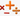## 任意二个数之和都是平方数

Tue, 26th November 2019Edit on Githubalgorithm平方数

2007年6月northwolves在CSDN提问 : 5个不同的自然数， 要求它们中的任意二个之和都是平方数

6个自然数有没有解？

# 详细内容

gxqcn给出一个预筛选思路。

1、要么全部为偶数，则可同除以最大公约数，必有奇数出现（进入下条款）；
2、有奇数，则最多允许出现一个；
2.1 若还有偶数。则奇数个数必为1，且偶数间关于 8 必同余；
2.2 若全为奇数。则奇数个数必为2，且其和被 4 整除。

mathe建议首先对于3个数和4个数的情况给出通解：

## 对于3个数的情况

$S=\frac{a^2+b^2+c^2}2$, 如果S为整数，那么$X=S-a^2,Y=S-b^2,Z=S-c^2$构成3个数的通解。

## 对于4个数的情况

N的奇素因子的数目不小于3

$a\lt b\lt c\lt d\lt e\lt f$ 使得
$a^2+f^2=b^2+e^2=c^2+d^2=N$
(这种分解可能不唯一，这时候，每个分解都可以对应一个4个数情况下的解）

$S=\frac{a^2+b^2+c^2}2$
$X=S-a^2$
$Y=S-b^2$
$Z=S-c^2$
$W=f^2-X$

（其中要求S是整数，如果S不是整数，我们将a,b,c,d,e,f全部乘上2就可以得到对于4N情况的一组解的）

mathe说上面方法可以求出4个数情况的所有解。

## 5个数的穷举方案

$\lt a,b,c;N\gt$
(可能还应该将数据$\lt a,c,e;N$\gt ; \lt a,b,d;N\gt\$也添加到这个表格中)

$\lt a,b,c;N\gt$
$\lt a,b,c;M\gt$

$N+M-(a^2+b^2+c^2)$

## 将整数N分解为平方数之和

$p_t=(u_t+i\times v_t)(u_t-i\times v_t)$ (其中$i^2=-1$)

$2=(1+i)(1-i)$

$p_t$$u_t+i\times v_t$$u_t-i\times v_t$来替换

## 素数表示为平方和的代码

mathe给出了下面的代码将一个素数p写成两个正整数平方和

#include <stdio.h>

#define abs(x) ((x)>0?(x):-(x))
#define HALFLIMIT 1000
#define LIMIT (HALFLIMIT*HALFLIMIT)
#ifdef WIN32
typedef __int64 longlong;
#else
typedef long long longlong;
#endif
int not_prime[LIMIT];

class cn{
longlong r,i;
public:
cn(longlong r, longlong i){this->r=r;this->i=i;}
cn com()const{cn x(r,-i);return x;}
longlong sqrmod()const{return r*r+i*i;}
longlong rel()const{return r;}
longlong img()const{return i;}
cn operator+(const cn& x)const{cn y(r+x.r,i+x.i);return y;}
cn operator-(const cn& x)const{cn y(r-x.r,i-x.i);return y;}
cn operator*(const cn& x)const{cn y(r*x.r-i*x.i,r*x.i+i*x.r);return y;}
cn div(longlong x)const;
bool iszero()const{return r==0&&i==0;}
};

cn cn::div(longlong x)const{
longlong u,v;
u=r%x;
v=i%x;
if(u<0)u+=x;
if(v<0)v+=x;
if(u>x/2)u-=x;
if(v>x/2)v-=x;
u=(r-u)/x;
v=(i-v)/x;
return cn(u,v);
}

cn round(const cn& x, const cn& y){
cn z=x*y.com();
longlong r=y.sqrmod();
return z.div(r);
}

cn factor(const cn& x, const cn& y){
if(x.iszero())return y;
return factor(y-round(y,x)*x,x);
}

int powmod(longlong a, longlong n, int p){
longlong mul=a%p;
longlong base=1;
while(n){
if(n&1){
base*=mul;
base%=p;
}
mul*=mul;
mul%=p;
n>>=1;
}
return (int)base;
}

int rm1(int p){
int i;
int t=(p-1)/4;
for(i=2;i<p;i++){
int u=powmod(i,t,p);
if(((longlong)u*u+1)%p==0)return u;
}
}

void defactor(int p){
int v=rm1(p);
cn x(v,1);
cn y(p,0);
cn f=factor(x,y);
printf("%d=%d^2+%d^2\n",p,abs((int)f.rel()),abs((int)f.img()));
}

int main(){
int i,j;
not_prime=not_prime=1;
for(i=2;i<HALFLIMIT;i++){
if(!not_prime[i]){
for(j=i*i;j<LIMIT;j+=i)
not_prime[j]=1;
}
}
for(i=2;i<LIMIT;i++){
if(!not_prime[i]&&i%4==1){
defactor(i);
}
}
}

## 更多的解

northwolves自己利用平方数末尾二位的特点：
00 01 04 09 16 21 24 25 29 36 41 44 49 56 61 64 69 76 81 84 89 96 做了一个很笨的循环，花了25分钟在 1000000内找到了http://www.iwr.uni-heidelberg.de/groups/ngg/People/winckler/PU/s051.html 提供的 两个解：

7442 28658 148583 177458 763442
32018 104882 188882 559343 956018

## 最终代码

mathe最后实现了他的上面介绍方案的代码 ,这个程序可以找到1483组5个数的解。

Github